# 一文看懂椭圆曲线签名算法## 1. 对称加密与非对称加密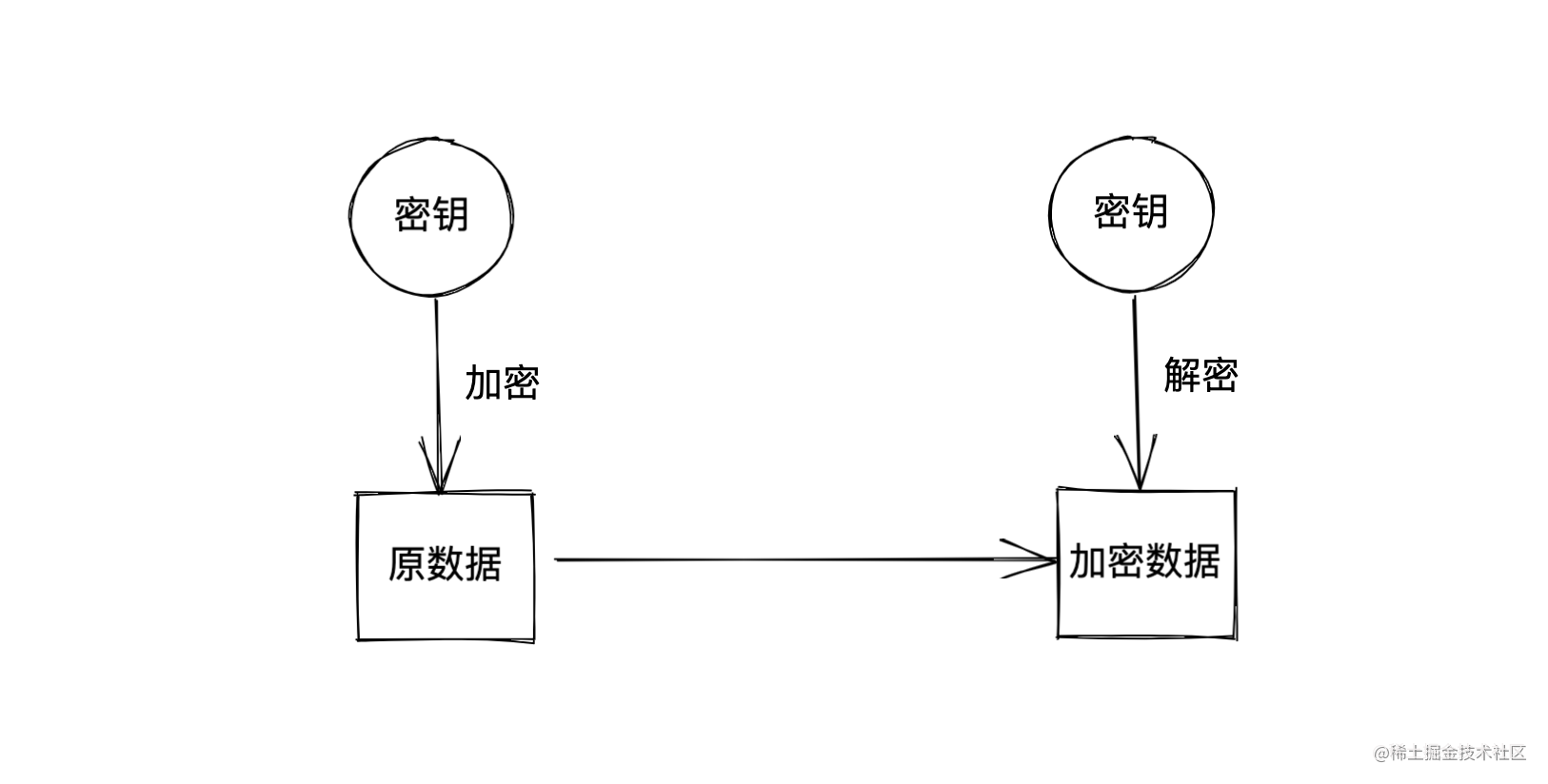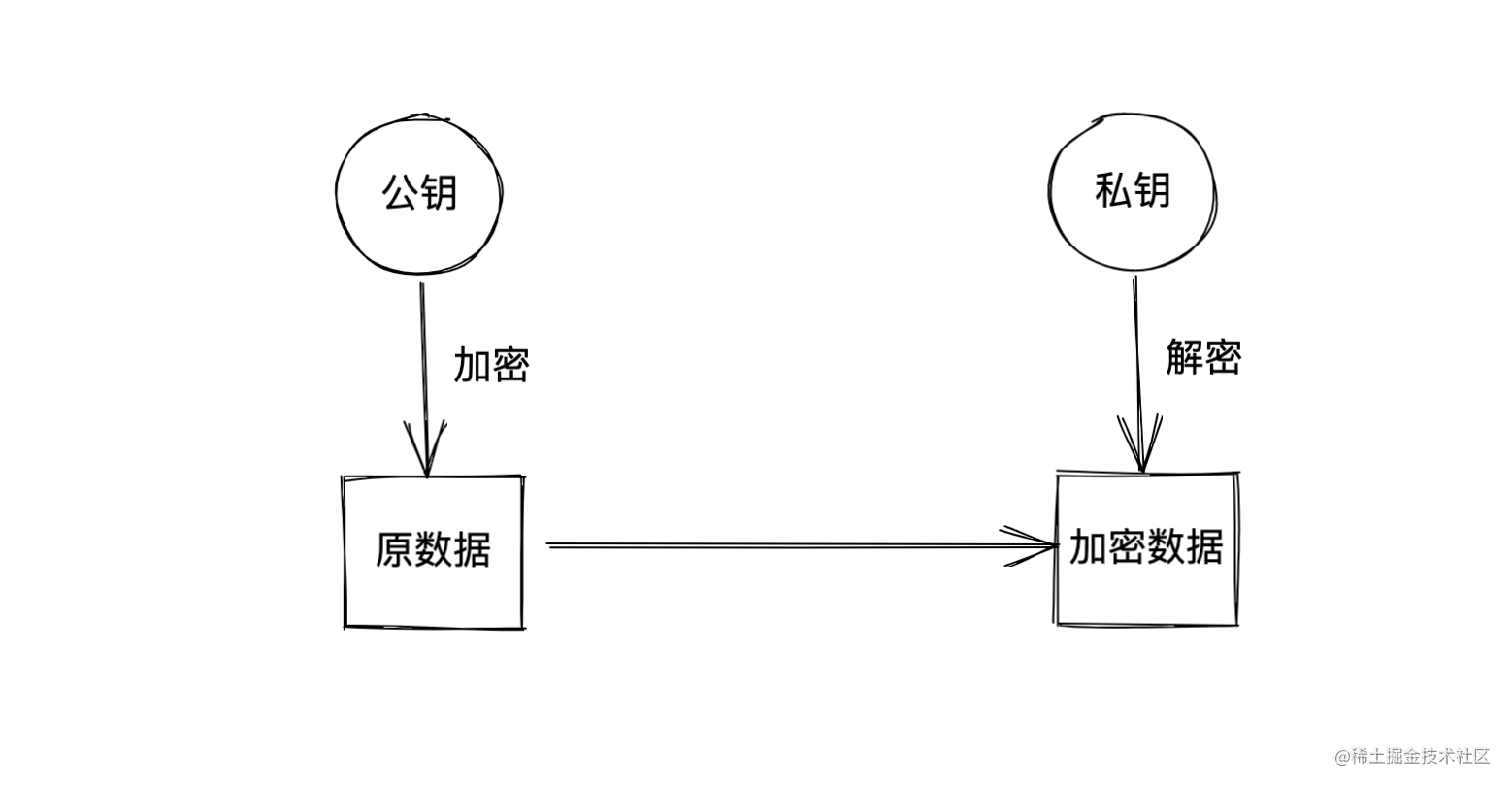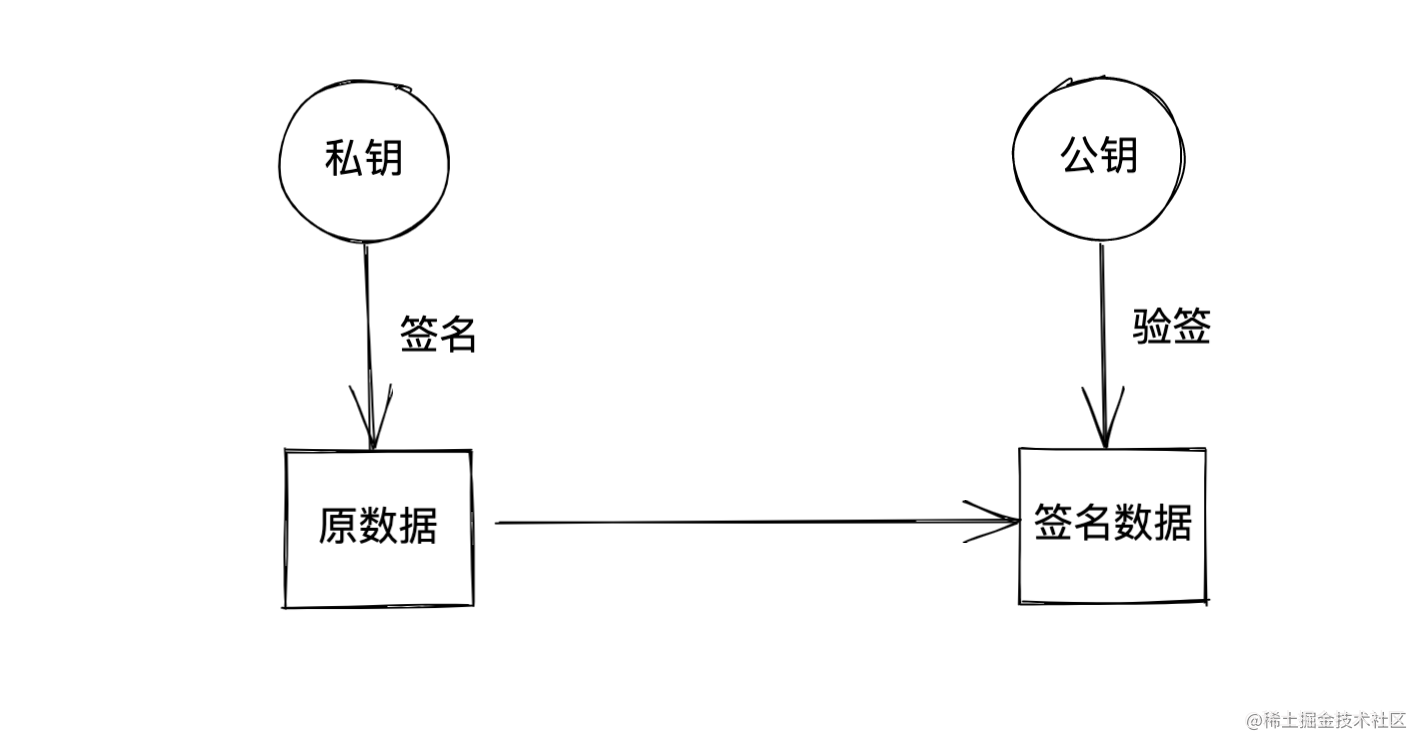RSA 和 ECC（Elliptic Curve Cryptography） 是两类比较出名的非对称加密算法，RSA 利用的是质数分解的原理来实现安全性。但是 RSA 对密钥长度的要求越来越高，这样也会造成计算量很大，而 ECC 使用的密钥长度则要短的多，而且安全性也更强。

## 2. 椭圆曲线算法

### 2.1 椭圆曲线的表示

$y^2 = (x^3 + a \times x + b)\quad mod\quad p$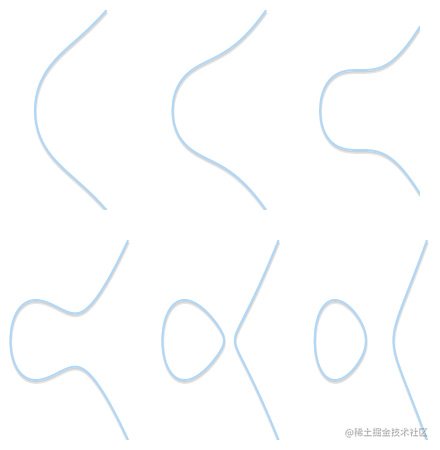### 2.2 椭圆曲线的运算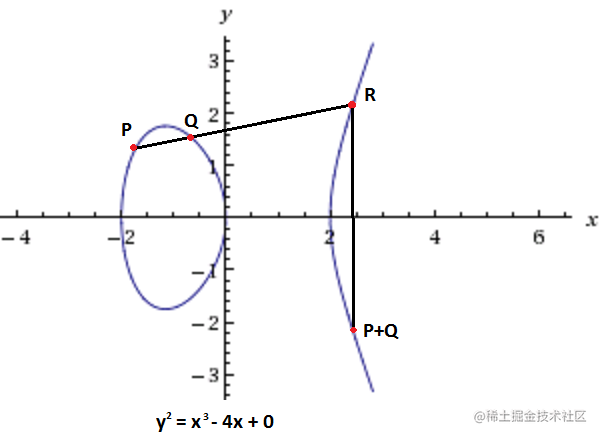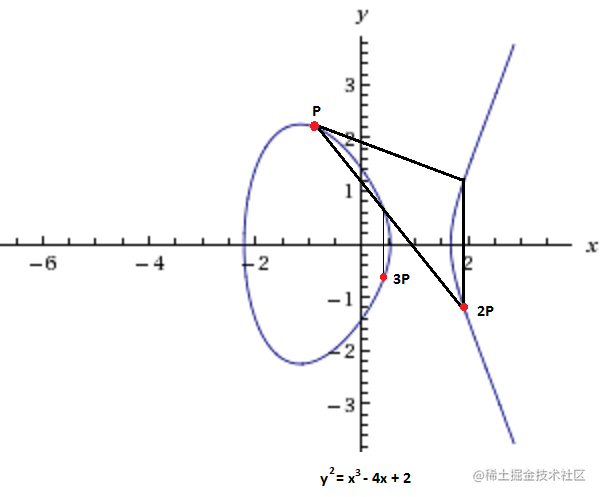## 3. 椭圆曲线签名算法

### 3.1 生成签名

1. 生成一个随机数 $k1$，注意这个随机数不是上面生成的私钥
2. 利用 $P = k1 \times G$ 计算点 $P1$，注意这个不是上面的公钥
3. $P1$ 点的 x 坐标就是 $R$
4. 对需要签名的数据计算 hash，为 $H$
5. 计算 $S = k1^{-1}(H + k0 \times R)\quad mod \quad p$，这个 $p$ 是模运算的底，也需要提前指定好

### 3.2 验证签名

$P = S^{-1} \times H \times G + S^{-1} \times R \times P0$

$P0$ 是公钥，通过这个公式，很容易就是可以计算得到 $P$， 得到 $P$ 之后，如果 $P$$x$ 坐标等于 $R$，那么就验签成功，在验签的过程中，完全不需要私钥的参与。